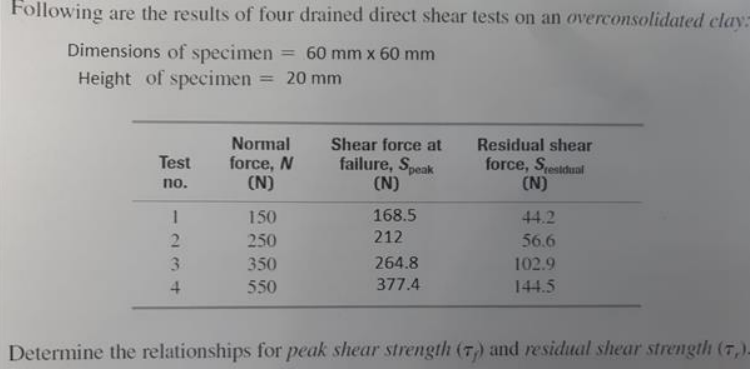Home / Expert Answers / Civil Engineering / direct-shear-test-data-is-given-in-the-figure-draw-the-shear-graph-show-your-calculations-and-ca-pa920

# (Solved): Direct shear test data is given in the Figure. Draw the shear graph, Show your calculations and ca ...Direct shear test data is given in the Figure. Draw the shear graph, Show your calculations and calculate cohesion and internal friction angle. (Shear box dimensions 60mmx60mm, thickness is 20mm,

Plot the shear stress versus effective normal stress Determine the cohesion and internal friciton angle ( for peak and residual) Hint: there should be 2 lines on the graph)

Following are the results of four drained direct shear tests on an overconsolidated clay: Dimensions of specimen $$=60 \mathrm{~mm} \times 60 \mathrm{~mm}$$ Height of specimen $$=20 \mathrm{~mm}$$ Determine the relationships for peak shear strength $$\left(\tau_{f}\right)$$ and residual shear strength $$\left(\tau_{r}\right)$$

We have an Answer from Expert